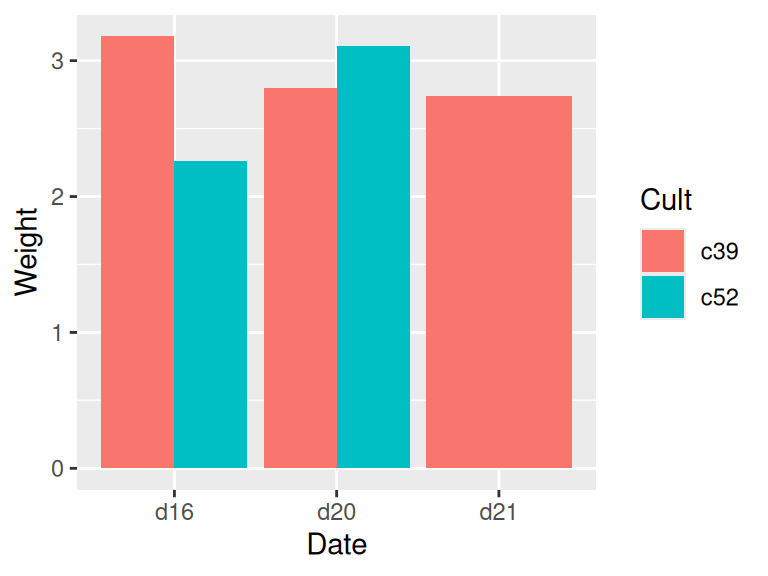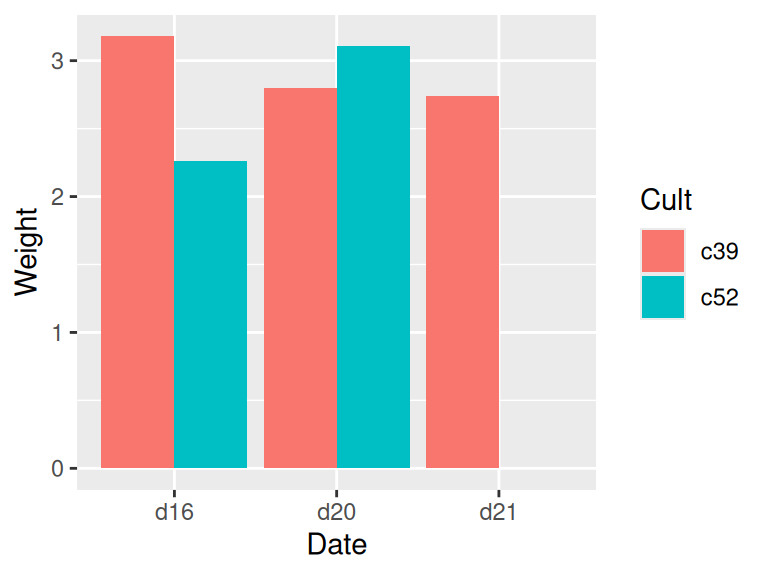## 15.17 Summarizing Data by Groups

### 15.17.1 Problem

You want to summarize your data, based on one or more grouping variables.

### 15.17.2 Solution

Use `group_by()` and `summarise()` from the dplyr package, and specify the operations to do:

``````library(MASS)  # Load MASS for the cabbages data set
library(dplyr)

cabbages %>%
group_by(Cult, Date) %>%
summarise(
VitC = mean(VitC)
)
#> `summarise()` has grouped output by 'Cult'. You can override using the
#> `.groups` argument.
#> # A tibble: 6 × 4
#> # Groups:   Cult 
#>   Cult  Date  Weight  VitC
#>   <fct> <fct>  <dbl> <dbl>
#> 1 c39   d16     3.18  50.3
#> 2 c39   d20     2.8   49.4
#> 3 c39   d21     2.74  54.8
#> 4 c52   d16     2.26  62.5
#> 5 c52   d20     3.11  58.9
#> 6 c52   d21     1.47  71.8``````

### 15.17.3 Discussion

There are few things going on here that may be unfamiliar if you’re new to dplyr and the tidyverse in general.

First, let’s take a closer look at the `cabbages` data set. It has two factors that can be used as grouping variables: `Cult`, which has levels `c39` and `c52`, and `Date`, which has levels `d16`, `d20`, and `d21`. It also has two numeric variables, `HeadWt` and `VitC`:

``````cabbages
#> 1   c39  d16    2.5   51
#> 2   c39  d16    2.2   55
#>  ...<56 more rows>...
#> 59  c52  d21    1.5   66
#> 60  c52  d21    1.6   72``````

Finding the overall mean of `HeadWt` is simple. We could just use the `mean()` function on that column, but for reasons that will soon become clear, we’ll use the `summarise()` function instead:

``````library(dplyr)
#>     Weight
#> 1 2.593333``````

The result is a data frame with one row and one column, named `Weight`.

Often we want to find information about each subset of the data, as specified by a grouping variable. For example, suppose we want to find the mean of each `Cult` group. To do this, we can use `summarise()` with `group_by()`.

``````tmp <- group_by(cabbages, Cult)
#> # A tibble: 2 × 2
#>   Cult  Weight
#>   <fct>  <dbl>
#> 1 c39     2.91
#> 2 c52     2.28``````

The command first groups the data frame `cabbages` based on the value of `Cult`. There are two levels of `Cult`, `c39` and `c52`, so there are two groups. It then applies the `summarise()` function to each of these data frames; it calculates `Weight` by taking the `mean()` of the `HeadWt` column in each of the sub-data frames. The resulting summaries for each group are assembled into a data frame, which is returned.

You can imagine that the `cabbages` data is split up into two separate data frames, then `summarise()` is called on each data frame (returning a one-row data frame for each), and then those results are combined together into a final data frame. This is actually how things worked in dplyr’s predecessor, plyr, with the `ddply()` function.

The syntax of the previous code used a temporary variable to store results. That’s a little verbose, so instead, we can use `%>%`, also known as the pipe operator, to chain the function calls together. The pipe operator simply takes what’s on its left and substitutes it as the first argument of the function call on the right. The following two lines of code are equivalent:

``````group_by(cabbages, Cult)
# The pipe operator moves `cabbages` to the first argument position of group_by()
cabbages %>% group_by(Cult)``````

The reason it’s called a pipe operator is that it lets you connect function calls together in sequence to form a pipeline of operations. Another common term for this is a different metaphor: chaining.

So the first argument of the function call is in a different place. So what? The advantages become apparent when chaining is involved. Here’s what it would look like if you wanted to call `group_by()` and then `summarise()` without making use of a temporary variable. Instead of proceeding left to right, the computation occurs from the inside out:

``summarise(group_by(cabbages, Cult), Weight = mean(HeadWt))``

Using a temporary variable, as we did earlier, makes it more readable, but a more elegant solution is to use the pipe operator:

``````cabbages %>%
group_by(Cult) %>%

Back to summarizing data. Summarizing the data frame by grouping using more variables (or columns) is simple: just give it the names of the additional variables. It’s also possible to get more than one summary value by specifying more calculated columns. Here we’ll summarize each `Cult` and `Date` group, getting the average of `HeadWt` and `VitC`:

``````cabbages %>%
group_by(Cult, Date) %>%
summarise(
Vitc = mean(VitC)
)
#> `summarise()` has grouped output by 'Cult'. You can override using the
#> `.groups` argument.
#> # A tibble: 6 × 4
#> # Groups:   Cult 
#>   Cult  Date  Weight  Vitc
#>   <fct> <fct>  <dbl> <dbl>
#> 1 c39   d16     3.18  50.3
#> 2 c39   d20     2.8   49.4
#> 3 c39   d21     2.74  54.8
#> 4 c52   d16     2.26  62.5
#> 5 c52   d20     3.11  58.9
#> 6 c52   d21     1.47  71.8``````

Note

You might have noticed that it says that the result is grouped by `Cult`, but not `Date`. This is because the `summarise()` function removes one level of grouping. This is typically what you want when the input has one grouping variable. When there are multiple grouping variables, this may or may not be the what you want. To remove all grouping, use `ungroup()`, and to add back the original grouping, use `group_by()` again.

It’s possible to do more than take the mean. You may, for example, want to compute the standard deviation and count of each group. To get the standard deviation, use `sd()`, and to get a count of rows in each group, use `n()`:

``````cabbages %>%
group_by(Cult, Date) %>%
summarise(
n = n()
)
#> `summarise()` has grouped output by 'Cult'. You can override using the
#> `.groups` argument.
#> # A tibble: 6 × 5
#> # Groups:   Cult 
#>   Cult  Date  Weight    sd     n
#>   <fct> <fct>  <dbl> <dbl> <int>
#> 1 c39   d16     3.18 0.957    10
#> 2 c39   d20     2.8  0.279    10
#> 3 c39   d21     2.74 0.983    10
#> 4 c52   d16     2.26 0.445    10
#> 5 c52   d20     3.11 0.791    10
#> 6 c52   d21     1.47 0.211    10``````

Other useful functions for generating summary statistics include `min()`, `max()`, and `median()`. The `n()` function is a special function that works only inside of the dplyr functions `summarise()`, `mutate()` and `filter()`. See `?summarise` for more useful functions.

The `n()` function gets a count of rows, but if you want to have it not count `NA` values from a column, you need to use a different technique. For example, if you want it to ignore any `NA`s in the `HeadWt` column, use `sum(!is.na(Headwt))`.

#### 15.17.3.1 Dealing with NAs

One potential pitfall is that `NA`s in the data will lead to `NA`s in the output. Let’s see what happens if we sprinkle a few `NA`s into `HeadWt`:

``````c1 <- cabbages # Make a copy
c1\$HeadWt[c(1, 20, 45)] <- NA # Set some values to NA

c1 %>%
group_by(Cult) %>%
summarise(
n = n()
)
#> # A tibble: 2 × 4
#>   Cult  Weight    sd     n
#>   <fct>  <dbl> <dbl> <int>
#> 1 c39       NA    NA    30
#> 2 c52       NA    NA    30``````

The problem is that `mean()` and `sd()` simply return `NA` if any of the input values are `NA.` Fortunately, these functions have an option to deal with this very issue: setting `na.rm=TRUE` will tell them to ignore the `NA`s.

``````c1 %>%
group_by(Cult) %>%
summarise(
Weight = mean(HeadWt, na.rm = TRUE),
sd = sd(HeadWt, na.rm = TRUE),
n = n()
)
#> # A tibble: 2 × 4
#>   Cult  Weight    sd     n
#>   <fct>  <dbl> <dbl> <int>
#> 1 c39     2.9  0.822    30
#> 2 c52     2.23 0.828    30``````

#### 15.17.3.2 Missing combinations {#_missing_combinations}

If there are any empty combinations of the grouping variables, they will not appear in the summarized data frame. These missing combinations can cause problems when making graphs. To illustrate, we’ll remove all entries that have levels `c52` and `d21`. The graph on the left in Figure 15.3 shows what happens when there’s a missing combination in a bar graph:

``````# Copy cabbages and remove all rows with both c52 and d21
c2 <- filter(cabbages, !( Cult == "c52" & Date == "d21" ))
c2a <- c2 %>%
group_by(Cult, Date) %>%

ggplot(c2a, aes(x = Date, fill = Cult, y = Weight)) +
geom_col(position = "dodge")``````

To fill in the missing combination (Figure 15.3, right), use the `complete()` function from the tidyr package – which is also part of the tidyverse. Also, the grouping for `c2a` must be removed, with `ungroup()`; otherwise it will return too many rows.

``````library(tidyr)
c2b <- c2a %>%
ungroup() %>%
complete(Cult, Date)

ggplot(c2b, aes(x = Date, fill = Cult, y = Weight)) +
geom_col(position = "dodge")``````
``````# Copy cabbages and remove all rows with both c52 and d21
c2 <- filter(cabbages, !( Cult == "c52" & Date == "d21" ))
c2a <- c2 %>%
group_by(Cult, Date) %>%
#> `summarise()` has grouped output by 'Cult'. You can override using the
#> `.groups` argument.

ggplot(c2a, aes(x = Date, fill = Cult, y = Weight)) +
geom_col(position = "dodge")
library(tidyr)
c2b <- c2a %>%
ungroup() %>%
complete(Cult, Date)

ggplot(c2b, aes(x = Date, fill = Cult, y = Weight)) +
geom_col(position = "dodge")``````Figure 15.3: Bar graph with a missing combination (left); With missing combination filled (right)

When we used `complete()`, it filled in the missing combinations with `NA`. It’s possible to fill with a different value, with the `fill` parameter. See `?complete` for more information.